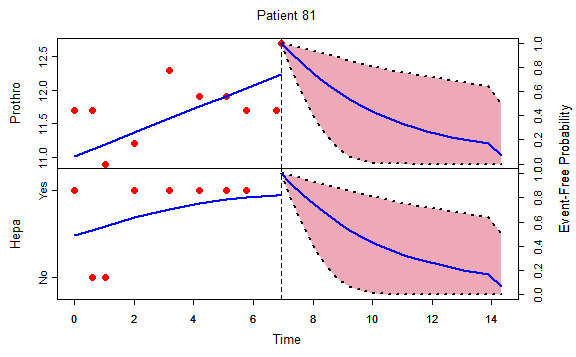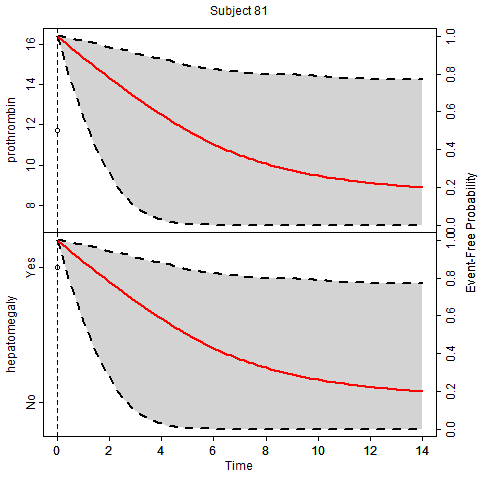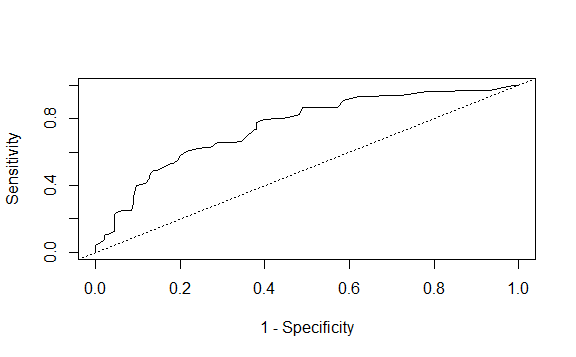# Joint Model Specification

We start with a general definition of the framework of multivariate joint models for multiple longitudinal outcomes and an event time. Let $$\mathcal D_n = \{T_i, \delta_i, y_i; i = 1, \ldots, n\}$$ denote a sample from the target population, where we let $$T_i^*$$ denote the true event time for the $$i$$-th subject, $$T_i$$ the observed event times, and $$\delta_i \in \{0, 1\}$$ denotes the event indicator, with 0 corresponding to right censoring and 1 to true events. Assuming $$K$$ longitudinal outcomes we let $$y_{ki}$$ denote the $$n_{ki} \times 1$$ longitudinal response vector for the $$k$$-th outcome ($$k = 1, \ldots, K$$) and the $$i$$-th subject, with elements $$y_{kij}$$ denoting the value of the $$k$$-th longitudinal outcome taken at time point $$t_{kij}$$, $$j = 1, \ldots, n_{ki}$$.

To accommodate multivariate longitudinal responses of different types in a unified framework, we postulate a generalized linear mixed effects model. In particular, the conditional distribution of $$y_{ki}$$ given a vector of random effects $$b_{ki}$$ is assumed to be a member of the exponential family, with linear predictor given by $g_k \bigl [ E \{ y_{ki}(t) \mid b_{ki} \} \bigr ] = \eta_{ki}(t) = x_{ki}^\top(t) \beta_k + z_{ki}^\top(t) b_{ki},$ where $$g_k(\cdot)$$ denotes a known one-to-one monotonic link function, and $$y_{ki}(t)$$ denotes the value of the $$k$$-th longitudinal outcome for the $$i$$-th subject at time point $$t$$, $$x_{ki}(t)$$ and $$z_{ki}(t)$$ denote the design vectors for the fixed-effects $$\beta_k$$ and for the random effects $$b_{ki}$$, respectively. The dimensionality and composition of these design vectors is allowed to differ between the multiple outcomes, and they may also contain a mix of baseline and time-varying covariates. To account for the association between the multiple longitudinal outcomes we link their corresponding random effects. More specifically, the complete vector of random effects $$b_i = (b_{1i}, b_{2i}, \ldots, b_{Ki})^\top$$ is assumed to follow a multivariate normal distribution with mean zero and variance-covariance matrix $$D$$. For the survival process, we assume that the risk for an event depends on a function of the subject-specific linear predictor $$\eta_i(t)$$ and/or the random effects. More specifically, we have $\begin{eqnarray} \nonumber h_i (t \mid \mathcal H_i(t), w_i(t)) & = & \lim_{\Delta t \rightarrow 0} \Pr \{ t \leq T_i^* < t + \Delta t \mid T_i^* \geq t, \mathcal H_i(t), w_i(t) \} \big / \Delta t, \quad t > 0\\ & = & h_0(t) \exp \biggl [\gamma^\top w_i(t) + \sum \limits_{k = 1}^K \sum \limits_{l = 1}^{L_k} f_{kl} \{\mathcal H_{ki}(t), w_i(t), b_{ki}, \alpha_{kl} \} \biggr], \end{eqnarray}$ where $$\mathcal H_{ki}(t) = \{ \eta_{ki}(s), 0 \leq s < t \}$$ denotes the history of the $$k$$-th outcome, and $$\mathcal H_i(t) = \{ H_{1i}(t), \ldots, H_{Ki}(t)\}$$ the history of the whole longitudinal process, $$h_0(\cdot)$$ denotes the baseline hazard function, $$w_i(t)$$ is a vector of exogenous, possibly time-varying, covariates with corresponding regression coefficients $$\gamma$$. Finally, the baseline hazard function $$h_0(\cdot)$$ is modeled flexibly using a B-splines approach, i.e., $\log h_0(t) = \sum \limits_{q = 1}^Q \gamma_{h_0,q} B_q(t, v),$ where $$B_q(t, v)$$ denotes the $$q$$-th basis function of a B-spline with knots $$v_1, \ldots, v_Q$$ and $$\gamma_{h_0}$$ the vector of spline coefficients.

# Dynamic Predictions

## Theory

Based on the general framework of joint models presented in the previous section, we are interested in deriving survival probabilities for a new subject $$j$$ that has survived up to time point $$t$$ and has provided longitudinal measurements $$\mathcal Y_{kj}(t) = \{ y_{kj}(t_{jl}); 0 \leq t_{jl} \leq t, l = 1, \ldots, n_j \}$$. The probabilities of interest are $\begin{array}{l} \pi_j(u \mid t) = \mbox{Pr}\{T_j^* > u \mid T_j^* > t, \mathcal Y_j(t), \mathcal D_n\}\\\\ = \displaystyle \int\int \frac{S\{u \mid \mathcal H_j(u, b_j), \theta\}}{S\{t \mid \mathcal H_j(t, b_j), \theta\}} \; p\{b_j \mid T_j^* > t, \mathcal Y_j(t), \theta\} \; p(\theta \mid \mathcal D_n) \; db_j d\theta, \end{array}$ where $$S(\cdot)$$ denotes the survival functional conditional on the random effects, and $$\mathcal Y_j(t) = \{\mathcal Y_{1j}(t), \ldots, \mathcal Y_{Kj}(t)\}$$. Combining the three terms in the integrand we can device a Monte Carlo scheme to obtain estimates of these probabilities, namely,

1. Sample a value $$\tilde \theta$$ from the posterior of the parameters $$[\theta \mid \mathcal D_n]$$.

2. Sample a value $$\tilde b_j$$ from the posterior of the random effects $$[b_j \mid T_j^* > t, \mathcal Y_j(t), \theta]$$.

3. Compute the ratio of survival probabilities $$S\{u \mid \mathcal H_j(u, \tilde b_j), \tilde \theta\} \Big / S\{t \mid \mathcal H_j(t, \tilde b_j), \tilde \theta\}$$.

Replicating these steps $$L$$ times, we can estimate the conditional survival probabilities by $\frac{1}{L} \sum_{l=1}^L \frac{S \bigl \{u \mid \mathcal H_j(u, \tilde b_j^{(l)}), \tilde \theta^{(l)} \bigr \}}{S \bigl \{t \mid \mathcal H_j(t, \tilde b_j^{(l)}), \tilde \theta^{(l)} \bigr \}},$ and their standard error by calculating the standard deviation across the Monte Carlo samples.

## Examples

We will illustrate the calculation of dynamic predictions using package JMbayes from a bivariate joint model fitted to the PBC dataset for the longitudinal outcomes prothrombin time (continuous) and hepatomegaly (dichotomous). We start by fit the bivariate mixed models using function mvglmer(). For both outcomes we only include the time effect in both the fixed and random effects. The code is:

library("JMbayes")
MixedModelFit <- mvglmer(list(prothrombin ~ year * sex + (year | id),
hepatomegaly ~ year * sex + (year | id)), data = pbc2,
families = list(gaussian, binomial))

Following, we fit the Cox model for the time to either transplantation or death. The first line defined the composite event indicator, and the second fits the Cox model in which we have also included the baseline covariates drug and age; note that we also need to set model = TRUE in the call to coxph(). The code is:

pbc2.id$event <- as.numeric(pbc2.id$status != "alive")
CoxFit <- coxph(Surv(years, event) ~ drug + age, data = pbc2.id, model = TRUE)

The joint model is fitted with the following call to mvJointModelBayes():

JMFit <- mvJointModelBayes(MixedModelFit, CoxFit, timeVar = "year")

We want to calculate predictions of the conditional survival probabilities for Patient 81. As first step, we extract the data of this patient in store them in the data.frame ND with the code:

ND <- pbc2[pbc2$id == 81, ] The calculation of the conditional survival probabilities is implemented in function survfitJM(). The basic/required arguments of this function are the fitted joint model, and the data of the patient(s) for whom we want the predictions. Hence, based on our fitted bivariate joint models and for Patient 81, we calculate the predictions by: sprobs <- survfitJM(JMFit, ND) sprobs ## ## Prediction of Conditional Probabilities of Event ## based on 200 Monte Carlo samples ## ##$81
##      times   Mean Median  Lower  Upper
## 1   6.9242 1.0000 1.0000 1.0000 1.0000
## 1   7.2140 0.9279 0.9432 0.8179 0.9824
## 2   7.6317 0.8308 0.8631 0.5862 0.9570
## 3   8.0495 0.7421 0.7849 0.3847 0.9313
## 4   8.4672 0.6617 0.7084 0.2279 0.9052
## 5   8.8849 0.5895 0.6339 0.1197 0.8788
## 6   9.3027 0.5251 0.5682 0.0550 0.8524
## 7   9.7204 0.4680 0.5042 0.0219 0.8263
## 8  10.1382 0.4177 0.4459 0.0075 0.8006
## 9  10.5559 0.3737 0.3924 0.0023 0.7764
## 10 10.9737 0.3351 0.3413 0.0007 0.7573
## 11 11.3914 0.3013 0.2916 0.0002 0.7391
## 12 11.8092 0.2715 0.2494 0.0000 0.7218
## 13 12.2269 0.2453 0.2124 0.0000 0.7051
## 14 12.6447 0.2221 0.1813 0.0000 0.6888
## 15 13.0624 0.2018 0.1543 0.0000 0.6729
## 16 13.4802 0.1838 0.1267 0.0000 0.6572
## 17 13.8979 0.1681 0.1038 0.0000 0.6416
## 18 14.3157 0.0683 0.0028 0.0000 0.5027

By default the function computed predictions starting from the time point of the last longitudinal measurements up to the end of the follow-up of the original dataset. Argument last.time can be used to indicate that the patient was event-free at a time point later than her last longitudinal measurement. In argument survTimes the user can provide her own time points at which she wishes to calculate the conditional survival probabilities.

We can depict the survival probabilities using the plot() method. The following call to the plot() method illustrates how many of the default arguments may be altered to fit the specific needs of the user.

plot(sprobs, split = c(2, 1), surv_in_all = TRUE,
lty_lines_CI = 3, col_lines = "blue", col_fill_CI = "pink2",
main = "Patient 81", ylab = c("Prothro", "Hepa"),
col_points = "red", pch_points = 16,
cex_xlab = 0.8, cex_ylab = 0.8, cex_zlab = 0.8,
cex_main = 0.8, cex_axis = 0.7)The most of the arguments are self-explaining given some background on the basic plot() method of R and of the options we have in par(). For example, lty_lines_CI is the lty (line type) option in par() for lines used to denote the 95% confidence interval, and col_points is the col (color) option in par() for the points denoting the observed longitudinal measurements. The split argument should be a vector of length 2 specifying in how many panels to split the figure (i.e., number of rows and columns). Argument surv_in_all specifies if the conditional survival function is to be included in all panels (i.e., a panel per longitudinal outcome).

To illustrate the concept of dynamic predictions, we calculate the conditional survival probabilities for Patient 81, starting from his first measurements and adding each time an extra measurement. We do this using a simple for-loop. The code is1:

N <- nrow(ND)
dyn_sprobs <- vector("list", N)
for (i in seq_len(N)) {
dyn_sprobs[[i]] <- survfitJM(JMFit, ND[1:i, ],
survTimes = seq(0, 14, length.out = 85))
plot(dyn_sprobs[[i]], split = c(2, 1), surv_in_all = TRUE)
}Dynamic Predictions

# Predictive Accuracy

## Discrimination and Calibration

Two general approaches have been proposed in the literature to assess predictive performance of survival models, namely, calibration, i.e., how well the model predicts the observed data, and discrimination, i.e., how well can the model discriminate between patients that had the event from patients that did not.

Discrimination: To take into account the dynamic nature of the longitudinal marker in discriminating between subjects, we focus on a time interval of medical relevance within which the occurrence of events is of interest. In this setting, a useful property of the model would be to successfully discriminate between patients who are going to experience the event within this time frame from patients who will not. To put this formally, as before, we assume that we have collected longitudinal measurements $$\mathcal Y_j(t)$$ up to time point $$t$$ for subject $$j$$. We are interested in events occurring in the medically-relevant time frame $$(t, t + \Delta t]$$ within which the physician can take an action to improve the survival chance of the patient. Under the assumed model and the methodology presented in the previous section, we can define a prediction rule using $$\pi_j(t + \Delta t \mid t)$$ that takes into account the available longitudinal measurements $$\mathcal Y_j(t)$$. In particular, for any value $$c$$ in $$[0, 1]$$ we can term subject $$j$$ as a case if $$\pi_j(t + \Delta t \mid t) \leq c$$ (i.e., occurrence of the event) and analogously as a control if $$\pi_j(t + \Delta t \mid t) > c$$. For a randomly chosen pair of subjects $$\{j, j'\}$$, in which both subjects have provided measurements up to time $$t$$, the discriminating capability of the assumed model can be assessed by the area under the receiver operating characteristic curve (AUC), which is obtained for varying $$c$$ and equals: $\mbox{AUC}(t, \Delta t) = \Pr \bigl [ \pi_j(t + \Delta t \mid t) < \pi_{j'}(t + \Delta t \mid t) \mid \{ T_j^* \in (t, t + \Delta t] \} \cap \{ T_{j'}^* > t + \Delta t \} \bigr ],$ that is, if subject $$j$$ experiences the event within the relevant time frame whereas subject $$j'$$ does not, then we would expect the assumed model to assign higher probability of surviving longer than $$t + \Delta t$$ for the subject who did not experience the event.

Calibration: The assessment of the accuracy of predictions of survival models is typically based on the expected error of predicting future events. In our setting, and again taking into account the dynamic nature of the longitudinal outcome, it is of interest to predict the occurrence of events at $$u > t$$ given the information we have recorded up to time $$t$$. This gives rise to expected prediction error: $\mbox{PE}(u \mid t) = E \bigl [ L\{N_i(u) - \pi_i(u \mid t)\} \bigr ],$ where $$N_i(t) = I(T_i^* > t)$$ is the event status at time $$t$$, $$L(\cdot)$$ denotes a loss function, such as the absolute or square loss, and the expectation is taken with respect to the distribution of the event times.

## Examples

We will illustrate how the above defined predictive accuracy measures can be calculated for the fitted bivariate joint model JMFit using the capabilities of package JMbayes. The functions that we will use are rocJM(), which calculates the ROC curve for different thresholds $$c$$ in $$[0, 1]$$, aucJM(), which calculates the area under the ROC curve, and prederrJM(), which calculates the prediction error. The required arguments are the fitted joint model, newdata a data.frame based on which to do the calculations, Tstart up to which time point we the patients are event-free (i.e., $$t$$ in the definitions of $$\mbox{AUC}(t, \Delta t)$$ and $$\mbox{PE}(u \mid t)$$), Dt the length of the medically relevant interval (i.e., $$\Delta t$$ in the definition of $$\mbox{AUC}(t, \Delta t)$$), and Thoriz at which time we want to predict (i.e., $$u$$ in the definition of $$\mbox{PE}(u \mid t)$$). To obtain objective estimates of the predictive accuracy measures we should calculate them in data that were not used to fit the model. However, in the following examples, and for the sake of simplicity, we calculate them in the PBC dataset what was also used to fit the model.

We focus on time point $$t = 5$$ years and we are interested in transplantations and deaths occurring within a $$\Delta t = 3$$-year interval. We start by calculating the ROC curve based on the bivariate joint model. The call to rocJM() is:

pbc2$event <- as.numeric(pbc2$status != "alive")
roc <- rocJM(JMFit, newdata = pbc2, Tstart = 5, Dt = 3)
roc
##
##  Time-dependent Sensitivity and Specificity for the Joint Model JMFit
##
## At time: 8
## Using information up to time: 5 (202 subjects still at risk)
##
##    cut-off         SN         SP        qSN         qSP    qOverall
## 1     0.25 0.02074299 1.00000000 0.01587106 1.000000000 0.031246214
## 2     0.30 0.04148598 1.00000000 0.03190084 1.000000000 0.061829266
## 3     0.35 0.10036613 0.97944324 0.06326783 0.480941885 0.111825083
## 4     0.40 0.18637322 0.95438534 0.11638381 0.424114859 0.182646536
## 5     0.45 0.25026582 0.92239517 0.14917806 0.346826011 0.208622610
## 6     0.50 0.39943496 0.90413209 0.27789204 0.430431858 0.337736978
## 7     0.55 0.48414082 0.86566162 0.34048384 0.383264726 0.360609889 *
## 8     0.60 0.55740267 0.80409678 0.38341613 0.305746477 0.340204560
## 9     0.65 0.63100874 0.72963517 0.42664435 0.241476458 0.308401012
## 10    0.70 0.66403339 0.65545712 0.41995508 0.181203973 0.253169369
## 11    0.75 0.79823998 0.59999204 0.60043605 0.191983925 0.290941857
## 12    0.80 0.82587952 0.51762357 0.60031434 0.145262823 0.233921746
## 13    0.85 0.91103535 0.41427082 0.73572264 0.117035112 0.201945703
## 14    0.90 0.96346872 0.21613017 0.78916233 0.051846072 0.097299782
## 15    0.95 1.00000000 0.01300467 1.00000000 0.003134714 0.006249837

The output of function gives a complete table of the sensitivity and specificity for each cut-off value $$c$$. The plot() method depicts the calculated ROC curve:

plot(roc)The area under the ROC curve is calculated using function aucJM() that has identical syntax as rocJM(), i.e.:

aucJM(JMFit, newdata = pbc2, Tstart = 5, Dt = 3)
##
##  Time-dependent AUC for the Joint Model JMFit
##
## Estimated AUC: 0.763
## At time: 8
## Using information up to time: 5 (202 subjects still at risk)

We observe that in the particular example, the combination of prothrobin time and hepatomegaly show respectable discrimination.

To evaluate how well we predict event at $$u = 8$$ years using information up to $$t = 5$$ years we use prederrJM():

prederrJM(JMFit, newdata = pbc2, Tstart = 5, Thoriz = 8)
##
## Prediction Error for the Joint Model JMFit
##
## Estimated prediction error: 0.1579
## At time: 8
## Using information up to time: 5 (202 subjects still at risk)
## Loss function: square

By default the square loss function is used, but the user can define her own loss function using argument lossFun.

1. The GIF was created using package animation.